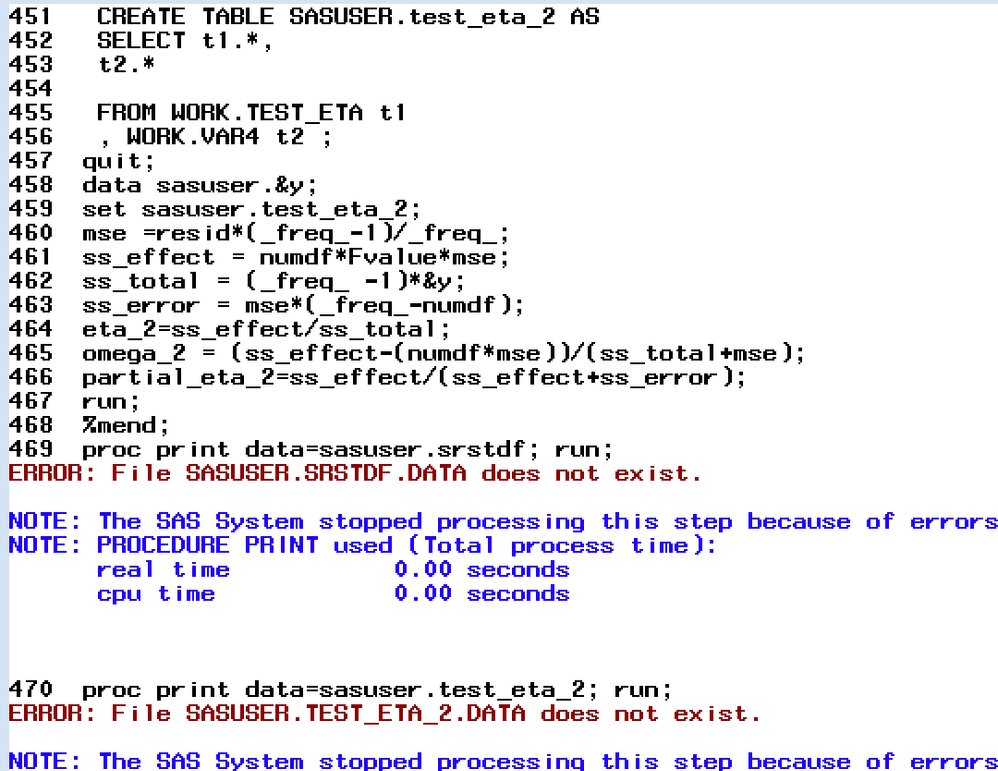## Running macro for proc MIXED? Results not computed/file not created

Hello SAS community,

I'm a student that's found themselves tasked with some advanced analysis to run in SAS -- thus far I've only been learning basic syntax by emulating the code of analyses ran by other colleagues, please bare with me and my novice exposure to SAS/macro code.

In short I've been trying to use macro code I found in academic paper to calculate pseudo effect size for a mixed model analysis -- I entered the components from my model into the macro as indicated (I included my original mixed model syntax below too) -- however, I am not getting any computations from the macro in my results, only results from the observations. The log shows the macro but no relevant errors, but if I add a PROC PRINT statements, it tells me files do not exist. I suspect that I'M not placing the macro in the correct DATA step but not sure. There's also univariate setup for the mixed model which uses ARRAY to create 'time', could my setup of this be reason why no results from macro?

Below is the full code I'm using and excerpt from error log, I'm using SAS 9.4 in Windows10

``````PROC IMPORT OUT= WORK.schizguat
DATAFILE= "\\Mac\Home\Desktop\SAS Files\CRC-2 r5 noid.sav"

DBMS=SPSS REPLACE;
RUN;

proc contents data=schizguat;
run;

data multidata ;
set schizguat;
keep SUBNUM DRUGCODEN  CRC2TOTALdiffV1MBAS CRC2TOTALdiffV2MBAS CRC2TOTALdiffV3MBAS BASELINECRC2TOTAL;
run;

proc print data=multidata (obs=42);
run;
*univariate set-up for mixed model;
data unidata;
set multidata;
array A(3)CRC2TOTALdiffV1MBAS--CRC2TOTALdiffV3MBAS;
subject +1;
do time=1 to 3;
srstdf=A(time);
output;
end;
drop CRC2TOTALdiffV1MBAS--CRC2TOTALdiffV3MBAS;
run;

data unidata;
set unidata;

run;

**** MACRO for Mixed Model Analysis with Effect Size ****;
%macro effectsize_rep(file=unidata,y=srstdf,class= SUBNUM DRUGCODEN time, fixed = BASELINECRC2TOTAL DRUGCODEN time time*DRUGCODEN); *NOTE: here is where I entered class/model items from my original mixed model;
TITLE1 "Mixed Models Analysis of: &Y";
PROC MIXED DATA = &file
PLOTS(ONLY)=ALL
METHOD=ML COVTEST; *NOTE: I changed syntax here (example I'm using had METHOD=REML) to be consistent with original mixed model;
CLASS &class;
MODEL &y =&fixed / outpm=outpm1 solution
ddfm=kr;
repeated time /subject=SUBNUM type=un r rcorr; *NOTE: I entered this REPEATED statement from original mixed model;
ods output tests3=tests3;
RUN; QUIT;
%_eg_conditional_dropds(var2);
PROC MEANS DATA=WORK.outpm1 nonobs noprint
FW=12
PRINTALLTYPES
CHARTYPE
VARDEF=N
VAR
N ;
VAR &y Resid;
OUTPUT OUT=var3
VAR()=
N()=
/ ;
RUN;
data var4;
set var3;
id=_n_;
run;
data test_eta;
set tests3;
id=_n_;
Run;
%_eg_conditional_dropds(SASUSER.QUERY_FOR_TEST_ETA);
%_eg_conditional_dropds(SASUSER.test_eta_2);
PROC SQL;
CREATE TABLE SASUSER.test_eta_2 AS
SELECT t1.*,
t2.*

FROM WORK.TEST_ETA t1
, WORK.VAR4 t2 ;
quit;
data sasuser.&y;
set sasuser.test_eta_2;
mse =resid*(_freq_-1)/_freq_;
ss_effect = numdf*Fvalue*mse;
ss_total = (_freq_ -1)*&y;
ss_error = mse*(_freq_-numdf);
eta_2=ss_effect/ss_total;
omega_2 = (ss_effect-(numdf*mse))/(ss_total+mse);
partial_eta_2=ss_effect/(ss_effect+ss_error);
run;
%mend;
proc print data=sasuser.srstdf; run;
proc print data=sasuser.test_eta_2; run;``````

Here is the syntax for original mixed model, these results run fine seperate from macro.

``````proc mixed data=unidata method=ml covtest;
class SUBNUM DRUGCODEN time;
model crctdf= BASELINECRC2TOTAL DRUGCODEN time  time*DRUGCODEN/solution;
repeated time /subject=SUBNUM type=un r rcorr;
lsmeans time*DRUGCODEN/diffs;
ods output diffs=drugdiffs lsmeans=druglsmeans;
title 'repeated measuresop  crc totoal diff  unstructured model baseline  cov';
run;``````

Error Log:I've been unsuccessful in finding solutions for correct macro setup for mixed model around the internet which tells me the solution may be obvious (my apologies if so) but there are so many complexities to the mixed model that I can't narrow down what it is. Any help is greatly appreciated, a million thanks in advance.

## Re: Running macro for proc MIXED? Results not computed/file not created

Please point to which line in the data is supposed to create that data set.

I didn't see anything that looked likely to create SASUSER.SRSDTF so I have to ask.

If it was supposed to be code not shown then more to be done.

It is easier to copy directly from the log and paste log entries into a code box, similar to the code, than to make a picture that we can't really copy or do much with directly.

You may need to run your macro with the system option MPRINT on to see the actual code generated by the macro. Then you may find the name of a data set is different than your Proc Print is attempting to use.

Discussion stats